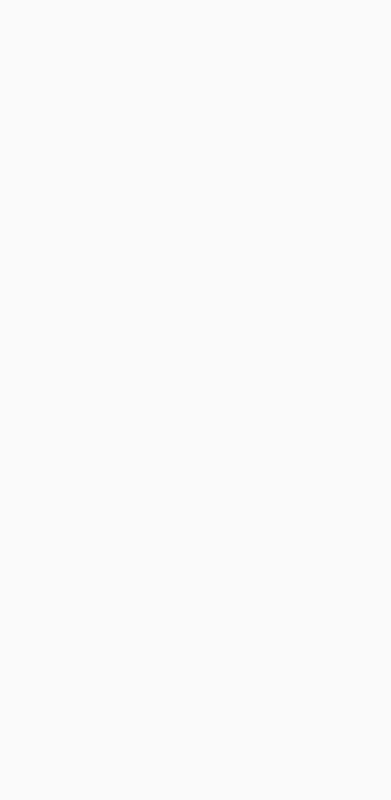# Split text lines in scanned document

From your input image, you need to make text as white, and background as blackYou need then to compute the rotation angle of your bill. A simple approach is to find the `minAreaRect` of all white points (`findNonZero`), and you get:Then you can rotate your bill, so that text is horizontal:Now you can compute horizontal projection (`reduce`). You can take the average value in each line. Apply a threshold `th` on the histogram to account for some noise in the image (here I used `0`, i.e. no noise). Lines with only background will have a value `>0`, text lines will have value `0` in the histogram. Then take the average bin coordinate of each continuous sequence of white bins in the histogram. That will be the `y` coordinate of your lines:Here the code. It’s in C++, but since most of the work is with OpenCV functions, it should be easy convertible to Python. At least, you can use this as a reference:

``````#include <opencv2/opencv.hpp>
using namespace cv;
using namespace std;

int main()
{

// Binarize image. Text is white, background is black
Mat1b bin;
cvtColor(img, bin, COLOR_BGR2GRAY);
bin = bin < 200;

// Find all white pixels
vector<Point> pts;
findNonZero(bin, pts);

// Get rotated rect of white pixels
RotatedRect box = minAreaRect(pts);
if (box.size.width > box.size.height)
{
swap(box.size.width, box.size.height);
box.angle += 90.f;
}

Point2f vertices;
box.points(vertices);

for (int i = 0; i < 4; ++i)
{
line(img, vertices[i], vertices[(i + 1) % 4], Scalar(0, 255, 0));
}

// Rotate the image according to the found angle
Mat1b rotated;
Mat M = getRotationMatrix2D(box.center, box.angle, 1.0);
warpAffine(bin, rotated, M, bin.size());

// Compute horizontal projections
Mat1f horProj;
reduce(rotated, horProj, 1, CV_REDUCE_AVG);

// Remove noise in histogram. White bins identify space lines, black bins identify text lines
float th = 0;
Mat1b hist = horProj <= th;

// Get mean coordinate of white white pixels groups
vector<int> ycoords;
int y = 0;
int count = 0;
bool isSpace = false;
for (int i = 0; i < rotated.rows; ++i)
{
if (!isSpace)
{
if (hist(i))
{
isSpace = true;
count = 1;
y = i;
}
}
else
{
if (!hist(i))
{
isSpace = false;
ycoords.push_back(y / count);
}
else
{
y += i;
count++;
}
}
}

// Draw line as final result
Mat3b result;
cvtColor(rotated, result, COLOR_GRAY2BGR);
for (int i = 0; i < ycoords.size(); ++i)
{
line(result, Point(0, ycoords[i]), Point(result.cols, ycoords[i]), Scalar(0, 255, 0));
}

return 0;
}
``````